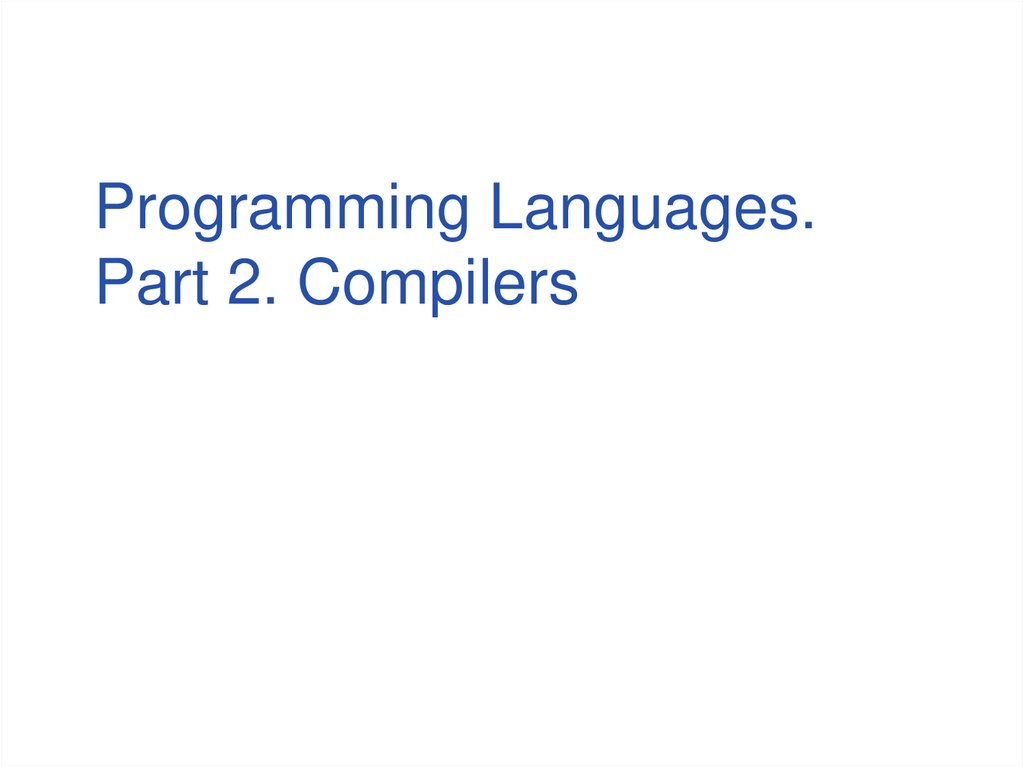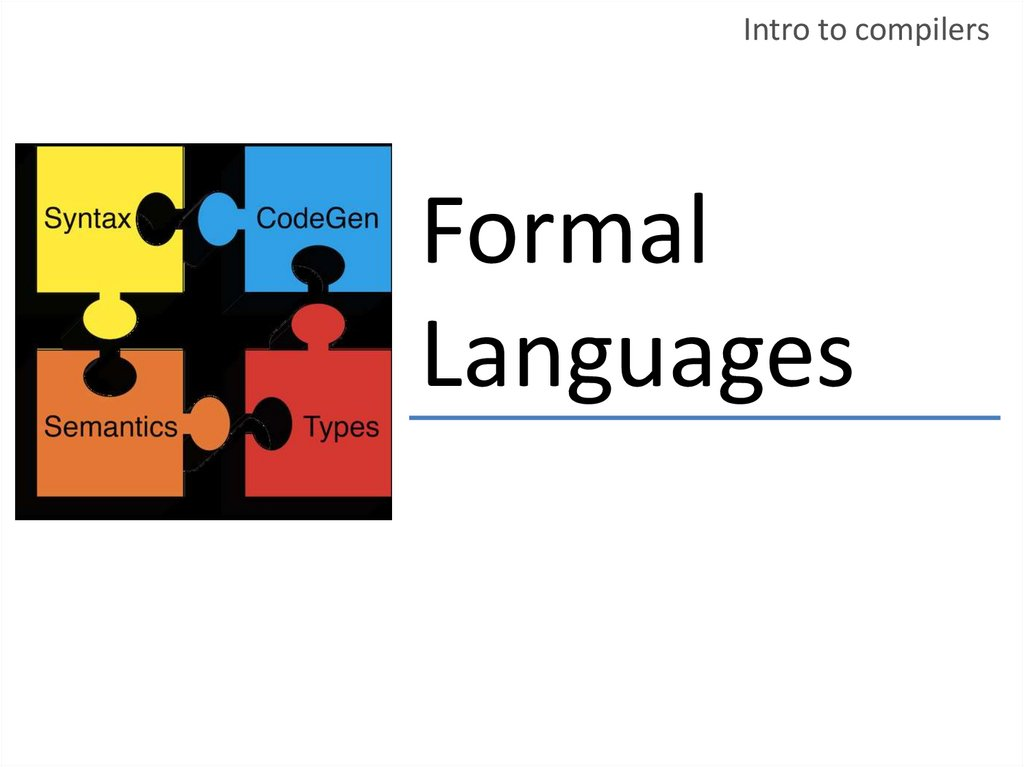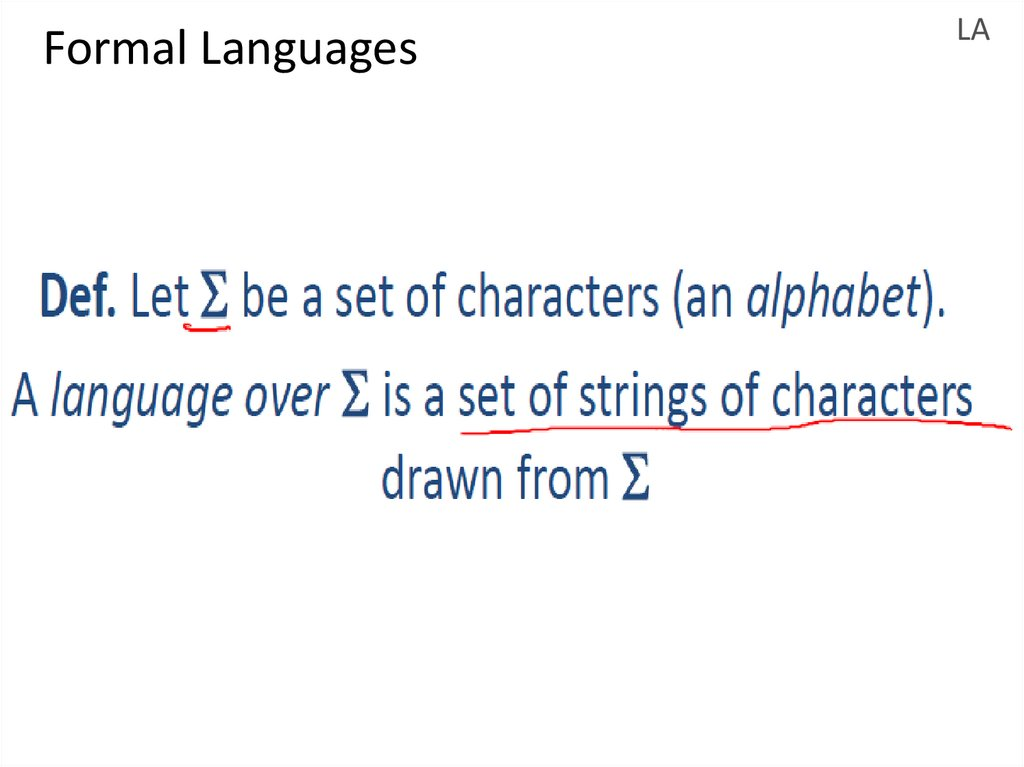# Programming Languages. Compilers. Formal Languages

## 2. Formal Languages

Intro to compilers
Formal
Languages

LA

## 4. Formal Languages

Intro to compilers
• Alphabet =
English
characters
• Alphabet = ASCII
• Language =
English
sentences
• Language = C
programs

## 5. Meaning function

Intro to compilers
Meaning function
Meaning function L maps syntax to
semantics
L(E) = Meanings
reg expr
set of strings

## 6. Meaning function

ɛ
= {“ “}
‘c’
= {“c“}
Intro to compilers
L : Exp -> Sets Strings

## 7. Meaning function

Intro to compilers
L( ɛ ) = { “ “ }
L(‘ c ’) = { “ c “ }
L(A + B) = L(A) U L(B)
L(AB) = {ab | a from L(A) ^ b from L(B)
L(A*) = U L(Ai)

## 8. Meaning function

• Why use a meaning function?
– Makes clear what is syntax, what is
semantics.
– Allows us to consider notation as a
separate issue
– Because expressions and meanings are
not 1-1
LA

LA
Meaning function
0
1
42
107
I
IV
X
XL

0*
0 + 0*
E + 00*
E + 0 + 0*
...
LA

## 11. Syntax and Semantic

• Meaning is many to one
– Never one to many!
LA